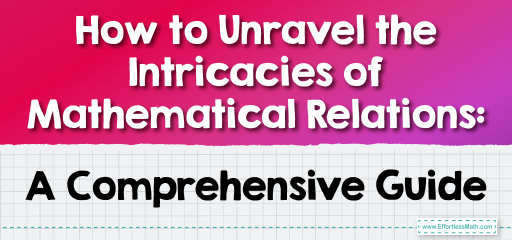# How to Unravel the Intricacies of Mathematical Relations: A Comprehensive Guide

Mathematics is a vast subject, so understanding "mathematical relations" can refer to a variety of concepts. Here, we provide a step-by-step guide to understanding the basic concept of a relation in mathematics, particularly in the context of sets and functions.## Step-by-step Guide to Understanding Mathematics Relations

Here is a step-by-step guide to understanding mathematics relations:

### Step 1: Introduction to Sets

• Definition: A set is a collection of distinct objects, considered as an object in its own right. For example, the numbers $$1, 2$$, and $$3$$ are distinct objects when considered separately, but when they are considered collectively as the set {$$1, 2, 3$$}, they form a single object.
• Notation: Sets are typically denoted with curly braces. For instance, {$$a, b, c$$} is a set with three elements.

### Step 2: Cartesian Product

• Before defining a relation, understand the Cartesian product.
• The Cartesian product of two sets, $$A$$ and $$B$$, denoted $$A×B$$, is the set of all ordered pairs $$(a,b)$$ where $$a$$ is in $$A$$ and $$b$$ is in $$B$$.
• Example: If $$A=$${$$1,2$$} and $$B=$${$$a,b$$}, then:

$$A×B=$${$$(1,a),(1,b),(2,a),(2,b)$$}.

### Step 3: Definition of a Relation

• A relation from set $$A$$ to set $$B$$ is a subset of the Cartesian product $$A×B$$.
• In other words, a relation is a set of ordered pairs where the first component of each pair comes from $$A$$ and the second component comes from $$B$$.

### Step 4: Visualizing Relations

• Relations can be represented using:
• a. Arrow Diagrams: Draw circles representing the sets, and arrows between the elements that are related.
• b. Graphs: Plot the relations on a coordinate plane.
• c. Matrices: Use $$1$$s and $$0$$s in a matrix to represent whether a relation exists between two elements or not.

### Step 5: Types of Relations (when both sets are the same, i.e., A = B):

• Reflexive: If every element is related to itself.
• Symmetric: If $$a$$ is related to $$b$$, then $$b$$ is related to $$a$$.
• Transitive: If $$a$$ is related to $$b$$ and $$b$$ is related to $$c$$, then $$a$$ is related to $$c$$.
• Antisymmetric: If $$a$$ is related to $$b$$ and $$b$$ is related to $$a$$, then $$a=b$$.

### Step 6: Special Relations – Functions

• A relation from set $$A$$ to set $$B$$ is called a function if every element of $$A$$ is related to exactly one element of $$B$$.
• Functions have special rules and properties, and they play a central role in many areas of mathematics.

### Step 7: Practice

• Try to create relations on small sets and check for their properties.
• Find real-world examples of relations. For example, the relation “is a parent of” on a set of people.

• For those interested in delving deeper, study “equivalence relations” and “partial orders,” which are special types of relations with unique properties.
• Relations also play a role in discrete mathematics, abstract algebra, and many other advanced fields.

Step 9: Conclusion

• A relation establishes a connection between elements of two sets. By understanding how elements relate to one another, you gain insight into patterns, structures, and properties within mathematics.

As you explore relations further, remember to use diagrams and real-world analogies to cement your understanding. The world of mathematical relations is vast and foundational to many advanced concepts, so take your time and build a solid understanding!

### Examples:

Example 1:

Consider the set $$P=$${$$4,5,6$$} and the relation $$Q$$ on $$P$$ defined as $$Q=$${$$(4,4),(5,5),(6,6)$$}. Is $$Q$$ reflexive?

Solution:

For a relation to be reflexive on a set, every element in the set must be related to itself.

From the relation $$Q$$:

• $$(4, 4)$$ is present, indicating $$4$$ is related to itself.
• $$(5, 5)$$ is present, indicating $$5$$ is related to itself.
• $$(6, 6)$$ is present, indicating $$6$$ is related to itself.

Since every element in $$P$$ is related to itself in $$Q$$, the relation $$Q$$ is reflexive on $$P$$.

Example 2:

Given the sets of ordered pairs,

$$R$$1$$​=$${$$(2,p),(4,z),(6,q)$$}

$$R$$2​$$=$${$$(4,q),(6,q),(2,p)$$}

are the same representation of relation $$R$$ from $$A=$${$$2,4,6$$} and $$B=$${$$p,q,r$$}. Find the value of $$z$$.

Solution:

To determine the value of $$z$$, we need to look at the ordered pair from set $$A$$ to set $$B$$ that involves the element $$4$$.

From $$R$$1​: The ordered pair with $$4$$ is $$(4, z)$$.

From $$R$$2​: The ordered pair with $$4$$ is $$(4, q)$$.

For​ $$R$$1 and $$R$$2 to represent the same relation, these ordered pairs must be equivalent. Therefore, $$z$$ must be the same as $$q$$.

So, $$z=q$$.

### What people say about "How to Unravel the Intricacies of Mathematical Relations: A Comprehensive Guide - Effortless Math: We Help Students Learn to LOVE Mathematics"?

No one replied yet.

X
30% OFF

Limited time only!

Save Over 30%

SAVE $5 It was$16.99 now it is \$11.99